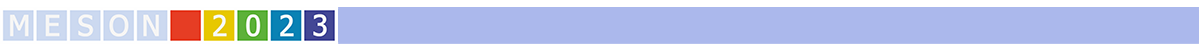#MESON2023

Jun 22 – 27, 2023
Auditorium Maximum
Europe/Warsaw timezone

## Precision measurements with Kaon decays at CERN

Jun 27, 2023, 11:30 AM
30m
Medium lecture hall (A and B) (Auditorium Maximum)

Plenary

### Speaker

Renato Fiorenza (Scuola Superiore Meridionale e INFN Sezione di Napoli)

### Description

The NA62 experiment at CERN collected the world's largest dataset of charged kaon decays in 2016-2018, leading to the first measurement of the branching ratio of the ultra-rare $K^+ \rightarrow \pi^+ \nu \bar\nu$ decay, based on 20 candidates. In this talk the NA62 experiment reports recent results from analyses of $K^+ \rightarrow \pi^0 e^+ \nu \gamma$, $K^+ \rightarrow \pi^+ \mu^+ \mu^-$ and $K^+\rightarrow \pi^+ \gamma \gamma$ decays, using a data sample recorded in 2017--2018. The radiative kaon decay $K^+ \rightarrow \pi^0 e^+ \nu \gamma$ (Ke3g) is studied with a data sample of O(100k) Ke3g candidates with sub-percent background contaminations. Preliminary results with the most precise measurements of the Ke3g branching ratios and T-asymmetry are presented. The $K^+ \rightarrow \pi^+ \mu^+ \mu^-$ sample comprises about 27k signal events with negligible background contamination, and the presented analysis results include the most precise determination of the branching ratio and the form factor. The $K^+ \rightarrow \pi^+ \gamma \gamma$ sample contains about 4k signal events with $10\%$ background contamination, and the analysis improves the precision of the branching ratio measurement by a factor of 3 with respect to the previous measurements.
Preliminary results of the $K^\pm \rightarrow \pi^0 \pi^0 \mu^\pm \nu$ (K00mu4) decay first observation and analysis based on the NA48/2 data collected in 2003-2004 are also presented.

Collaboration NA62, NA48/2

### Primary author

Angela Romano (University of Birmingham)